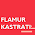Saturday, April 9, 2016

Structures deform when subjected to various conditions such as external loads, change in temperature, differential settlement, or fabrication errors. Deformation is often considered in terms of rotation(slope) or translation(deflection). Slope is the angle (in radians) which the elastic curve makes with the original neutral axis of the member, while deflection is the movement the structural member makes from the original neutral axis. In design of structures, it is necessary to control the deflection for the purpose of aesthetics and appropriate functionality (like cracking of finishes).

In beams and frames, bending moment is the major cause of deflection, while in trusses, axial forces are the major cause. Deformation due to shear is often very small and is neglected in most simple analysis. In the solved example that is downloadable, the frame shown above is completely analysed, and the horizontal deflection at point B is obtained using the virtual work method. The analysis was carried out assuming linear elastic material response. Both the integration method (Mohr's integral) and graphical method (Vereschagin's diagram combination method) were used in the analysis.

The steps involved in the analysis of the frame can be summarised as follows:

(1) Analyse the frame completely and draw the final bending moment diagram due to externally

(2) Remove the externally applied load, and replace it with a virtual unit load, in the direction that the deflection is sought. For slopes, you place a unit moment at the point you wish to obtain the rotation.

(3) Analyse the frame completely under the action of the virtual load and draw the virtual bending moment diagram.

(4) Combine the bending moment equations or diagrams of the external and virtual loads and compute the value of the deflection.

Download the final solved example here and feel free to ask questions.

1.2.